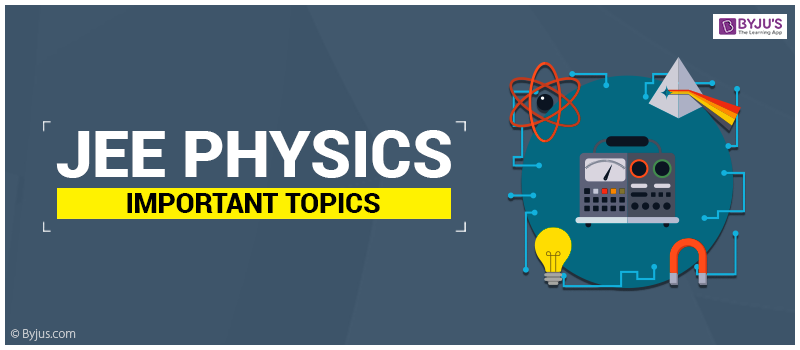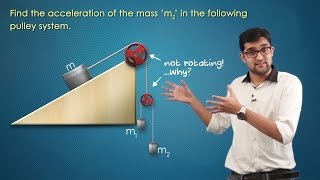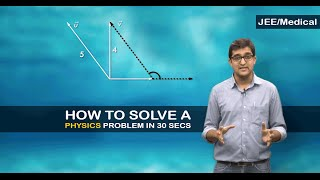# JEE Physics Important Topics JEE Main And AdvancedMost of the JEE aspirants consider physics as one of their favourite subjects because it not only helps them to develop critical thinking but also helps them to acquire problem-solving skills. It also happens to be a key subject particularly in highly competitive examinations like JEE Main and Advanced. In JEE exams, the questions asked in the physics section are generally based on the conceptual knowledge about the topic. For scoring well, you are required to have a strong conceptual base along with the practical application of concepts.

There are around 20 chapters included in JEE Physics syllabus and out of these, there are several important topics that must be focused on while preparing for JEE Physics:

1. Mechanics: The questions asked from this unit are either directly based on some mechanical concepts or might require considerable skills in mechanics, which could help you in solving the question. JEE mechanics is all about few fundamental laws like – Newton’s laws of motion, laws of gravitation, laws of conservation of linear and angular momentum. All these laws form a framework in physics and thus it is expected that you should understand all these laws and apply them to practical problems.

Questions from mechanics could be solved easily by the combination of Laws of conservation of energy, Newton’s laws, Equation of continuity, Work energy theorem, Laws of conservation of momentum and Bernoulli’s theorem. These topics have applications in other chapters of Physics too. Therefore, they are probably the most important topics that you need to focus on.

2. Electricity and magnetism: Due to its vastness and the level of difficulty, this unit is very crucial for both JEE Main and Advanced. Every year lots of questions are asked in JEE from this unit. You don’t have to worry much about physics in JEE if you practice and prepare well for this unit. You can expect lots of questions from capacitance, coulombs law, and its applications.

Don’t get scared of complicated circuit problems and try to understand the fundamentals for reducing complicated electrical circuits into simpler ones. Practice several questions from Kirchhoff’s laws and series-parallel circuit reduction and also don’t ignore the theoretical aspects of the topic. Memorise all the formulas and practice several questions from electrostatics, Motional EMF, and magnetostatics.

3. Fluid mechanics and Thermal physics: Familiarize yourself with the calculations of work, heat, Carnot engine and its efficiency, internal energy and the laws of thermodynamics. Several times in JEE Exams questions from the laws of thermodynamics were mixed with the calorimetry. Practice several numbers of questions from fluid mechanics as well.

4. Oscillation and waves: Every year around 4 to 5 questions are generally asked from this unit. Try to understand certain complex topics like the principle of superposition, reflection & refraction of waves, forced and damped oscillations, resonance, Simple Harmonic Motion, Doppler effects in sound through visualization. It will not only make you understand the concepts easily but will also help you in retaining it for a longer period of time.

5. Optics: This is one of the easiest and high scoring unit. Practice several numbers of questions from this unit and try to focus more on certain important topics like Interference, Young’s double slit experiment, wavefront, Huygens’ principle, Laws of reflection & refraction, Polarisation, Dispersion of light by the prism, Lens Formula and Magnification.

6. Modern physics: Mostly theoretical questions are asked from this unit. Focus more on understanding the Bohr’s model of atomic structure. Formula-based questions are generally asked from this unit, therefore, memorize all the formulas properly and use them correctly while answering the questions.

### IIT JEE Physics Important Topics

We will take a look at JEE 2019 physics section that includes JEE Main paper and JEE Advanced paper.

## JEE Main 2019 Physics Section

During JEE Main 2019, the physics section was deemed to be the toughest section among the three sections. The questions were tricky, lengthy and students had to make an extra effort to solve the questions with their logical and reasoning skills. The physics section consisted of a total of 30 marks. In this, 13 questions were set from electromagnetism, 8 from mechanics 2 from modern physics, 3 from optics, 3 from thermodynamics, 1 from SHM and Waves. In this paper, 10 questions were quite easy, 17 were moderate and 3 questions were difficult.

 Physics Topics No of Questions Weightage of Marks Electromagnetics Induction; AC 1 4 Solids and Fluids 1 4 Waves 1 4 Work, Power and Energy 1 4 Gravitation 1 4 Simple Harmonic Motion 1 4 Unit, Dimension and Vector 1 4 Kinematics 1 4 Laws of motion 1 4 Centre Of Mass, Impulse and Momentum 1 4 Rotation 1 4 Magnetics 2 8 Heat and Thermodynamics 3 12 Current Electricity 3 12 Electrostatics 3 12 Optics 3 12 Modern Physics 5 20 Total 30 120

Apart from this, some of the important chapters for 2019 include;

• Ray Optics
• Electrostatics
• Photoelectric Effect and Electromagnetic Wave
• Current Electricity

## JEE Main 2018 Physics Section

In 2018 JEE Main question paper the physics section, as usual, was difficult. Most of the questions were designed to test the conceptual knowledge. As for the number, there were 13 questions were from the class 11th syllabus and 17 questions were from class 12th syllabus. In this section, there were 20 moderate, 6 easy questions and 4 difficult questions.

 Topics Marks Weightage No. of Questions Mechanics 40 10 Thermodynamics 8 2 Optics 8 2 Modern Physics 24 6 Electrodynamics 36 9 SHM & Waves 4 1 Total 120 30

### JEE Advanced 2018 Physics Section

In JEE Advanced paper 1, the physics section was on a moderate level and consisted of 11 easy questions, 5 were moderate and 2 were difficult. In paper 2, there were seven MCQs with a single correct answer. The total weightage for this section was 21 marks where 9 questions each were asked from both the 11th and 12th standard syllabus.
 Physics Topics No of Questions Weightage of Marks Mechanics 15 41.67% Electrodynamics 8 22.50% Heat & Thermodynamics 4 10.83% SHM & Waves 3 8.33% KTG & Thermodynamics 3 8.33% Error in Measurement 3 7.50% Electrostatics 3 8.33% Rigid Body Dynamics 3 8.33% Modern Physics 3 8.33% Elasticity & Viscosity 2 5.83% Unit & Dimension 2 5.00% Electromagnetic Field 2 5.83% Optics 2 5.83% Geometrical Optics & Physical Optics 2 5.83% Sound Waves 2 5.83% Gravitation 1 2.50% Surface Tension 1 3.33% Work, Power & Energy 1 3.33% Heat Transfer 1 2.50% Circular Motion 1 3.33% Mathematical Tool 1 2.50% Current Electricity 1 2.50% Nuclear Physics 1 3.33% Photoelectric Effect 1 2.50% Bohr Atomic Model 1 2.50% Electromagnetic Induction 1 3.33% Capacitance 1 2.50% SHM 1 2.50% Mathematical Tools 1 2.50% Projectile Motion 1 2.50%

To know more about IIT JEE chemistry and maths topics as well as be in touch with the latest JEE Main and JEE Advanced notifications and updates keep visiting BYJU’S.

#### Pulley Problems#### Solve JEE Problem